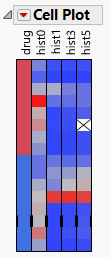Essential Graphing > Cell Plots
Publication date: 05/24/2021

# Cell Plots

##### View Color-Intensity Plots of Variables

Using cell plots, you can visualize each cell in a data table. Cell plots are direct representations of a data table, since they draw a rectangular array of cells where each cell corresponds to a data table entry. Cell plots were popularized by genomics applications to browse large numbers of values for gene expression levels.

Figure 8.1 Example of a Cell Plot